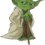# Gravitational Fields

October 28, 2023To begin with, the speed of gravity has not been measured directly in the laboratory—the gravitational interaction is too weak, and such an experiment is beyond present technological capabilities. The "speed of gravity" must therefore be deduced from astronomical observations, and the answer depends on what model of gravity one uses to describe those observations.

In the simple newtonian model, gravity propagates instantaneously: the force exerted by a massive object points directly toward that object's present position. For example, even though the Sun is 500 light seconds from the Earth, newtonian gravity describes a force on Earth directed towards the Sun's position "now, " not its position 500 seconds ago. Putting a "light travel delay" (technically called "retardation") into newtonian gravity would make orbits unstable, leading to predictions that clearly contradict Solar System observations.

In general relativity, on the other hand, gravity propagates at the speed of light; that is, the motion of a massive object creates a distortion in the curvature of spacetime that moves outward at light speed. This might seem to contradict the Solar System observations described above, but remember that general relativity is conceptually very different from newtonian gravity, so a direct comparison is not so simple. Strictly speaking, gravity is not a "force" in general relativity, and a description in terms of speed and direction can be tricky. For weak fields, though, one can describe the theory in a sort of newtonian language. In that case, one finds that the "force" in GR is not quite central—it does not point directly towards the source of the gravitational field—and that it depends on velocity as well as position. The net result is that the effect of propagation delay is almost exactly cancelled, and general relativity very nearly reproduces the newtonian result.

This cancellation may seem less strange if one notes that a similar effect occurs in electromagnetism. If a charged particle is moving at a constant velocity, it exerts a force that points toward its present position, not its retarded position, even though electromagnetic interactions certainly move at the speed of light. Here, as in general relativity, subtleties in the nature of the interaction "conspire" to disguise the effect of propagation delay. It should be emphasized that in both electromagnetism and general relativity, this effect is not put in ad hoc but comes out of the equations. Also, the cancellation is nearly exact only for constant velocities. If a charged particle or a gravitating mass suddenly accelerates, the change in the electric or gravitational field propagates outward at the speed of light.

Since this point can be confusing, it's worth exploring a little further, in a slightly more technical manner. Consider two bodies—call them A and B—held in orbit by either electrical or gravitational attraction. As long as the force on A points directly towards B and vice versa, a stable orbit is possible. If the force on A points instead towards the retarded (propagation-time-delayed) position of B, on the other hand, the effect is to add a new component of force in the direction of A's motion, causing instability of the orbit. This instability, in turn, leads to a change in the mechanical angular momentum of the A-B system. But total angular momentum is conserved, so this change can only occur if some of the angular momentum of the A-B system is carried away by electromagnetic or gravitational radiation.

Now, in electrodynamics, a charge moving at a constant velocity does not radiate. Technically, the lowest-order radiation is dipole radiation, and the radiated power depends on the second time derivative of the electric dipole moment; two time derivatives give acceleration. So, to the extent that A's motion can be approximated as motion at a constant velocity, A cannot lose angular momentum. For the theory to be consistent, there must therefore be compensating terms that partially cancel the instability of the orbit caused by retardation. This is exactly what happens; a calculation shows that the force on A points not towards B's retarded position, but towards B's "linearly extrapolated" retarded position.

In general relativity, roughly speaking, a mass moving at a constant acceleration does not radiate. Here, the lowest order radiation is quadrupole radiation, and the radiated power depends on the third time derivative of the mass quadrupole moment. (The full picture is slightly more complex, since one cannot have a single, isolated accelerating mass; whatever it is that causes the acceleration also has a gravitational field, and its field must be taken into account.) For consistency, just as in the case of electromagnetism, a cancellation of the effect of retardation must occur, but it must now be even more complete—that is, it must hold to a higher power of /c. This is exactly what one finds when one solves the equations of motion in general relativity.

While current observations do not yet provide a direct model-independent measurement of the speed of gravity, a test within the framework of general relativity can be made by observing the binary pulsar PSR 1913+16. The orbit of this binary system is gradually decaying, and this behavior is attributed to the loss of energy due to escaping gravitational radiation. But in any field theory, radiation is intimately related to the finite velocity of field propagation, and the orbital changes due to gravitational radiation can equivalently be viewed as damping caused by the finite propagation speed. (In the discussion above, this damping represents a failure of the "retardation" and "noncentral, velocity-dependent" effects to completely cancel.)

Source: math.ucr.edu
##### INTERESTING VIDEOGravitational Fields - A Level PhysicsMagnetic Gravitational Fields viewA2 Gravitational Fields

##### INTERESTING FACTS##### What does the gravitational field strength on a planet depend on.

It can be calculated on the basis of the planet's mass and its radius.